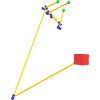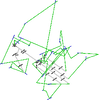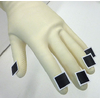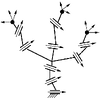Kinematic Synthesis of Tree Topologies

Kinematic synthesis consists of the theoretical design of robots to comply with a given task. In this project we focus on finite point kinematic synthesis, that is, given a specific robotic topology and a task defined by spatial positions, we design a robot with that topology that complies with the task.

Tree topologies consist of loop-free structures where there can be many end-effectors. A characteristic of these topologies is that there are many shared joints. This allows some structures that may seem redundant to not actually be redundant when considering all the end-effectors at once. The main focus of this work is the design of grippers that have topologies similar to that of the human hand, which can be seen as a tree topology.

This research was developed in collaboration with Dr. Alba Perez-Gracia.

Overview

In this research we investigated the kinematic synthesis of tree articulated structures. In particular these structures are interesting as they are found in natural life forms. A straight-forward example would be the case of human hands that can be modelled using revolute joints. In order to be able to perform kinematic synthesis of these structures, we must understand the entire process in depth and establish criteria in which the system of equations does not degenerate. For this purpose we have presented both practical applications of this work and the more theoretical aspects of these structures.

We start by defining the system of equations needed to solve for tree topologies using Clifford algebra. An approach to solve the equations numerically using a hybrid optimizer based on Levenberg-Marquadt local optimizer and genetic algorithms was presented. We show that this approach is able to consistently find solutions despite the complexity of these equations that make them unsolvable by traditional methods.

We later extend this formulation to take into account velocities, accelerations and further derivatives using Lie algebra. We show that this simplifies the equation system and allows more control in the definition of tasks. By defining the proper velocity and derivatives at points in space it is possible to approximate arbitrary curves. Additionally the same optimization framework can be used.

Finally we do a more in-depth theoretical analysis of the conditions needed in order to be able to perform finite point kinematic synthesis on tree topologies. This is not as straight-forward as it seems as there may be sub-topologies that become overdetermined for certain tasks. This causes the equations to generate and not have any solutions. In order to avoid this problem, the topology must be analyzed in depth. We propose representing the topology as a compacted tree graph, and present the necessary criteria for the design equations to be solvable for a given task.

Full source code for all the publications is available.

Publications

2014Kinematic Synthesis using Tree Topologies
Edgar Simo-Serra, Alba Perez-Gracia
Mechanism and Machine Theory (MAMT) 72:94-113, 2014
This paper presents a methodology for the description and finite-position dimensional synthesis of articulated systems with multiple end-effectors. The articulated system is represented as a rooted tree graph. Graph and dimensional synthesis theories are applied to determine when exact finite-position synthesis can be performed on the tree structures by considering the motion for all the possible subgraphs. Several examples of tree topologies are presented and synthesized. This theory has an immediate application on the design of novel multi-fingered hands.
@Article{SimoSerraMAMT2014,
author    = {Edgar Simo-Serra and Alba Perez-Gracia},
title     = {{Kinematic Synthesis using Tree Topologies}},
journal   = {Mechanism and Machine Theory},
volume    = {72},
pages     = {94--113},
year      = 2014,
}

2012Kinematic Synthesis of Multi-Fingered Robotic Hands for Finite and Infinitesimal Tasks
Edgar Simo-Serra, Alba Perez-Gracia, Hyosang Moon, Nina Robson
Advances in Robot Kinematics (ARK), 2012
In this paper we present a novel method of designing multi-fingered robotic hands using tasks composed of both finite and infinitesimal motion. The method is based on representing the robotic hands as a kinematic chain with a tree topology. We represent finite motion using Clifford algebra and infinitesimal motion using Lie algebra to perform finite dimensional kinematic synthesis of the multi-fingered mechanism. This allows tasks to be defined not only by displacements, but also by the velocity and acceleration at different positions for the design of robotic hands. An example task is provided using an experimental motion capture system and we present the design of a robotic hand for the task using a hybrid Genetic Algorithm/Levenberg-Marquadt solver.
@InProceedings{SimoSerraARK2012,
author    = {Edgar Simo-Serra and Alba Perez-Gracia and Hyosang Moon and Nina Robson},
title     = {{Kinematic Synthesis of Multi-Fingered Robotic Hands for Finite and Infinitesimal Tasks}},
booktitle = "Advances in Robot Kinematics (ARK)",
year      = 2012,
}

2011Design of Non-Anthropomorphic Robotic Hands for Anthropomorphic Tasks
Edgar Simo-Serra, Francesc Moreno-Noguer, Alba Perez-Gracia
ASME International Design Engineering Technical Conferences (IDETC), 2011
In this paper, we explore the idea of designing non-anthropomorphic, multi-fingered robotic hands for tasks that replicate the motion of the human hand. Taking as input data rigid-body trajectories for the five fingertips, we develop a method to perform dimensional synthesis for a kinematic chain with a tree structure, with three common joints and five branches. We state the forward kinematics equations of relative displacements for each serial chain expressed as dual quaternions, and solve for up to five chains simultaneously to reach a number of poses along the hand trajectory using a hybrid global numerical solver that integrates a genetic algorithm and a Levenberg-Marquardt local optimizer. Although the number of candidate solutions in this problem is very high, the use of the genetic algorithm lets us to perform an exhaustive exploration of the solution space and retain a subset of them. We then can choose some of the solutions based on the specific task to perform. Note that these designs could match the task exactly while having a finger design radically different from that of the human hand.
@InProceedings{SimoSerraIDETC2011,
author    = {Edgar Simo-Serra and Francesc Moreno-Noguer and Alba Perez-Gracia},
title     = {{Design of Non-Anthropomorphic Robotic Hands for Anthropomorphic Tasks}},
booktitle = "Proceedings of the 2011 ASME International Design Engineering Technical Conferences",
year      = 2011,
}Kinematic Model of the Hand using Computer Vision
Edgar Simo-Serra
Degree Thesis, 2011
Biotechnology is a science that is growing rapidly. The objective of this project is to advance in the field. Specifically it aims to study applications of kinematics in the field of human-machine interfaces namely exoskeleton and prosthesis designs for the human hand. The methodology used in this project consists of three phases. First a theoretical model of the hand kinematics is defined from medical literature. This is done by synthesizing the hand into its simplified parameters to define a robotic model. The adjustment of the theoretical model to a hand is then done by capturing the movement using computer vision. This is done by using markers on each nail to be able to estimate their poses which consist of their spatial orientation and position. This sequence permits estimating the movement of the entire hand. Dimensional kinematic synthesis is finally applied to adapt the theoretical model to the dataset provided by computer vision. This is done by defining the equations of the movement of the theoretical hand model and is then solved by a numerical solver. This allows the creation of a personalized hand model that can then be used for the study of correspondences between electromyography and the movements of the hand. In conclusion, this project has designed a robust algorithm for the tracking and estimation of the poses of the nails of the hand. It has also defined the movement equations and created an application to solve them. This has led to the finding of many non-anthropomorphic models that can be of use in the design of exoskeletons.
@mastersthesis{SimoSerraPFC2011,
author    = {Edgar Simo-Serra},
title     = {{Kinematic Model of the Hand using Computer Vision}},
school    = "BarcelonaTech (UPC)",
type      = "Degree Thesis",
year      = 2011,
}

Source Codelibdq, 2.2 (Feb, 2013)
Library for using and manipulating unit dual quaternions to describe rigid body motions
This is a library for using and manipulating unit dual quaternions (Clifford algebra Cl^+(0,3,1). Unit dual quaternions are useful for describing rigid body movements using screw theory. Main applications of using unit dual quaternions are found in kinematics. The core of the library is written in C. However, a Lua interface is also provided which is installable by means of LuaRocks. This provides a simple interface for the quick prototyping of projects.ArtTreeKS, 1.0 (Mar, 2012)
Kinematic synthesis solver for tree topologies
ArtTreeKS is a dedicated kinematic synthesis solver for tree topologies. It is designed specifically for finite position kinematic synthesis and has support for both position constraints as well as velocity or acceleration constraints. The solver employed is a hybrid solved that is based on a Genetic Algorithm (GA) built on top of a Levenberg-Marquadt local optimizer. This allows obtaining solutions for very complex tree topologies.